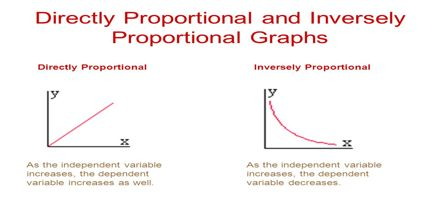Mathematic

# Directly and Inversely ProportionalGeneral objective of this lecture is to present on Directly and Inversely Proportional. Example like; weight(w) is directly proportional to mass(m) and directly proportional to acceleration due to gravity(g). Pressure(P) is directly proportional to the Force(F)and inversely proportional to the area(A). Frequency(f) is inversely proportional to the time(t). Acceleration(a) is directly proportional to the Force(F) and inversely proportional to the mass(m).